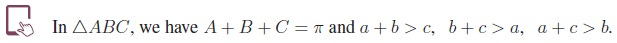Home | | Maths 11th std | Properties of Triangle

# Properties of Triangle

One important use of trigonometry is to solve practical problems that can be modeled by a triangle.

Properties of Triangle

One important use of trigonometry is to solve practical problems that can be modeled by a triangle. Determination of all the sides and angles of a triangle is referred as solving the triangle. In any triangle, the three sides and three angles are called basic elements of a triangle. Pythagorean theorem plays a vital role in finding solution of the right triangle. The law of sines and the law of cosines are important tools that can be used effectively in solving an oblique triangle ( a triangle with no right angle). In this section, we shall discuss the relationship between the sides and angles of a triangle and derive the law of sines and the law of cosines.Notation:  Let ABC be a triangle. The angles of  ŌłåABC corresponding to the vertices A, B, C are denoted by A, B, C them selves. The sides opposite to the angles A, B, C are denoted by a, b, c respectively. Also we use the symbol Ōłå to denote the area of a triangle.

The circle which passes through the three vertices of a triangle is called circumcircle of the triangle. The centre and the radius R of the circumcircle are known as circumcentre and circumradius respectively.Tags : Trigonometry | Mathematics , 11th Mathematics : UNIT 3 : Trigonometry
Study Material, Lecturing Notes, Assignment, Reference, Wiki description explanation, brief detail
11th Mathematics : UNIT 3 : Trigonometry : Properties of Triangle | Trigonometry | Mathematics